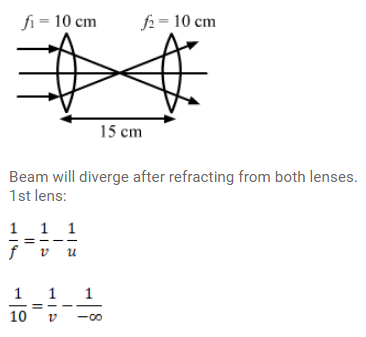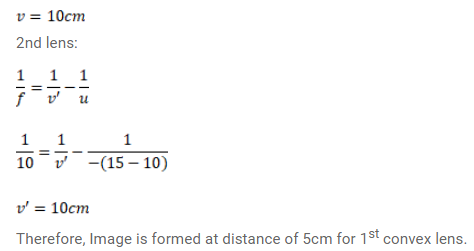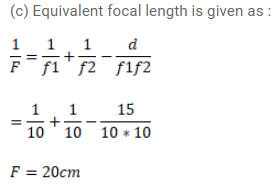# Two convex lenses, each of focal lengthQuestion:

Two convex lenses, each of focal length $10 \mathrm{~cm}$, are placed at a separation of $15 \mathrm{~cm}$ with their principal axes coinciding

(a) show that a light beam coming parallel to the principal axis diverges as it comes out of the lens system.

(b) Find the location of the virtual image formed by the lens system of an object placed far away.

(c) Find the focal length of the equivalent lens. (note that the sign of the focal length is positive although the lens system actually diverges a parallel beam incident on it.)

Solution: